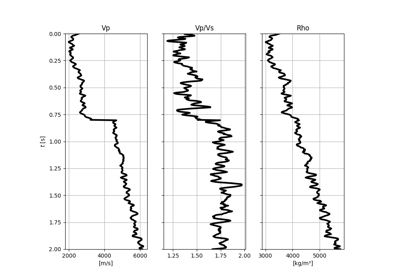# pylops.avo.avo.AVOLinearModelling¶

class pylops.avo.avo.AVOLinearModelling(theta, vsvp=0.5, nt0=1, spatdims=None, linearization='akirich', dtype='float64')[source]

AVO Linearized modelling.

Create operator to be applied to a combination of elastic parameters for generation of seismic pre-stack reflectivity.

Parameters: theta : np.ndarray Incident angles in degrees vsvp : np.ndarray or float VS/VP ratio nt0 : int, optional number of samples (if vsvp is a scalar) spatdims : int or tuple, optional Number of samples along spatial axis (or axes) (None if only one dimension is available) linearization : str, optional choice of linearization, akirich: Aki-Richards, fatti: Fatti dtype : str, optional Type of elements in input array. NotImplementedError If linearization is not an implemented linearization

Notes

The AVO linearized operator performs a linear combination of three (or two) elastic parameters arranged in input vector $$\mathbf{m}$$ of size $$n_{t0} \times N$$ to create the so-called seismic reflectivity:

$r(t, \theta, x, y) = \sum_{i=1}^N G_i(t, \theta) m_i(t, x, y) \qquad \forall \quad t, \theta$

where $$N=2/3$$. Note that the reflectivity can be in 1d, 2d or 3d and spatdims contains the dimensions of the spatial axis (or axes) $$x$$ and $$y$$.

Attributes: shape : tuple Operator shape explicit : bool Operator contains a matrix that can be solved explicitly (True) or not (False)

Methods

 __init__(self, theta[, vsvp, nt0, spatdims, …]) Initialize this LinearOperator. adjoint(self) Hermitian adjoint. apply_columns(self, cols) Apply subset of columns of operator cond(self, \*\*kwargs_eig) Condition number of linear operator. conj(self) Complex conjugate operator div(self, y[, niter]) Solve the linear problem $$\mathbf{y}=\mathbf{A}\mathbf{x}$$. dot(self, x) Matrix-matrix or matrix-vector multiplication. eigs(self[, neigs, symmetric, niter]) Most significant eigenvalues of linear operator. matmat(self, X) Matrix-matrix multiplication. matvec(self, x) Matrix-vector multiplication. rmatmat(self, X) Adjoint matrix-matrix multiplication. rmatvec(self, x) Adjoint matrix-vector multiplication. todense(self) Return dense matrix. transpose(self) Transpose this linear operator.

## Examples using pylops.avo.avo.AVOLinearModelling¶AVO modelling